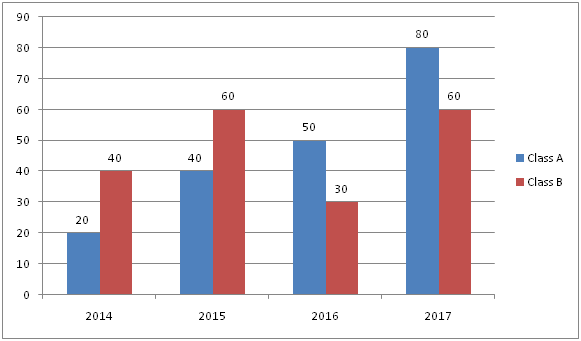# NIACL AO Prelims – Quantitative Aptitude Questions Day- 75

Dear Readers, Bank Exam Race for the Year 2019 is already started, To enrich your preparation here we have providing new series of Practice Questions on Quantitative Aptitude – Section. Candidates those who are preparing for NIACL AO Prelims 2019 Exams can practice these questions daily and make your preparation effective.

[WpProQuiz 4861]

1) Bus A and Bus B apart from 50km. If it’s travel towards each other, then meet after one hour. Bus A running faster than bus B. Find the speed of bus A, if its overtakes B after 5 hours running in the direction of bus B?

a) 30 kmph

b) 40 kmph

c) 20 kmph

d) 28 kmph

e) None of these

2) A gave 60% of the amount he had to B. B in turn gave 25% of what he received from A to C. C bought a mobile at Rs.6000 out of the amount he got from B and Rs.3000 is left from C. How much amount did A have?

a) Rs.60000

b) Rs.50000

c) Rs.40000

d) Rs.20000

e) None of these

3) Rudra, Sunil and Sakthi started a business with total investment is Rs.24000. Sunil invested Rs.3000 more than Sakthi and Sakthi invested Rs.1500 less than Rudra. If the total profit at the end of year is Rs.48000, then find the sum of the share received by Sunil and Sakthi?

a) Rs.32000

b) Rs.28000

c) Rs.30000

d) Rs.26000

e) None of these

4) A milk man mixes the two types of milk, one is Rs.20 per litre and other one is Rs.30 per litre in the ratio of 2:3. If he sells the mixture at Rs.28 per litre, find the approximate profit percentage?

a) 8%

b) 6%

c) 4%

d) 12%

e) 10%

5) If Rs.20000 is divided into two parts such that the SI on the first part for 8 years at 10% per annum is equal to the SI on the second part for 8 years at 15% per annum, find the difference between the larger amount and smaller amount?

a) Rs.4000

b) Rs.5000

c) Rs.3000

d) Rs.6000

e) None of these

Directions (6-10): Study the following information carefully and answer the given questions.

The given graph shows the number of students in the Class A and Class B.6) If in the year 2018, there is 25% increase in the total number of students in 2017 in both classes, then find the total number of students in 2018?

a) 175

b) 160

c) 180

d) 170

e) None of these

7) Find the difference between the average number of students in class A at first three years together and the average number of students in class B at last three years together?

a) 40/3

b) 44/3

c) 52/3

d) 50/3

e) None of these

8) The sum of the students in class A and class B in 2015 and 2016 together is approximately what percent of the sum of the students in class A and class B in 2014 and 2017 together?

a) 80%

b) 90%

c) 70%

d) 60%

e) None of these

9) What is the ratio of the total number of students in class A in all the given years together to the total number of students in class B in all the given years together?

a) 2: 5

b) 3: 2

c) 1: 2

d) 2: 3

e) None of these

10) The number of students of class B in 2017 is approximately what percent of the total number of students of class A in all the years together?

a) 32%

b) 28%

c) 35%

d) 39%

e) 42%

Speed of bus A=x kmph

Speed of bus B=y kmph

X + y =50 —- (1)

5x – 5y =50

X – y =10 —– (2)

Solve the equation (1) and (2), we get

2x = 60

X = 30 kmph

A=Rs. x

B=60/100*x=3x/5

C=3x/5*25/100=3x/20

3x/20-6000=3000

3x/20=9000

x=60000

Rudra investment=x

Sakthi=x-1500

Sunil=x-1500+3000=x+1500

X+x-1500+x+1500=24000

3x=24000

X=8000

Rudra=8000

Sakthi=6500

Sunil=9500

Profit ratio=8000:6500:9500

=16:13:19

Sum of Sakthi and Sunil=32/48*48000=Rs.32000

Total cost= (2x*20+3x*30) =Rs.130x

SP=5x*28=140x

Profit=140x-130x=10x

Required percentage=10x/130x*100=8%

X*8*10/100= (20000-x)*8*15/100

2x=(20000-x)3

2x = 60000 – 3x

5x = 60000

= > x = 12000

Another part=20000-12000=8000

Difference=12000-8000=Rs.4000

Direction (6-10) :

Class A=80*125/100=100

Class B=60*125/100=75

Total=100+75=175

Average class A= (20+40+50)/3=110/3

Average class B= (60+30+60)/3=150/3=50

Difference=50-(110/3) =40/3

Sum in 2015 and 2016= (40+50) + (60+30) =180

Sum in 2014 and 2017= (20+80) + (40+60) =200

Required percentage=180/200*100=90%

Required ratio= (20+40+50+80): (40+60+30+60)

=190:190=1: 1

Required percentage=60/190*100=32%